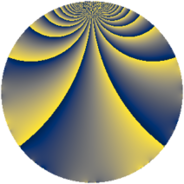# Properties

 Label 1425.2.bLevel $1425$ Weight $2$ Character orbit 1425.b Rep. character $\chi_{1425}(1424,\cdot)$ Character field $\Q$ Dimension $116$ Sturm bound $400$

# Related objects

## Defining parameters

 Level: $$N$$ $$=$$ $$1425 = 3 \cdot 5^{2} \cdot 19$$ Weight: $$k$$ $$=$$ $$2$$ Character orbit: $$[\chi]$$ $$=$$ 1425.b (of order $$2$$ and degree $$1$$) Character conductor: $$\operatorname{cond}(\chi)$$ $$=$$ $$285$$ Character field: $$\Q$$ Sturm bound: $$400$$

## Dimensions

The following table gives the dimensions of various subspaces of $$M_{2}(1425, [\chi])$$.

Total New Old
Modular forms 212 124 88
Cusp forms 188 116 72
Eisenstein series 24 8 16

## Trace form

 $$116q - 96q^{4} - 12q^{6} + 4q^{9} + O(q^{10})$$ $$116q - 96q^{4} - 12q^{6} + 4q^{9} + 72q^{16} + 14q^{19} + 28q^{24} - 44q^{36} - 8q^{39} - 96q^{49} - 40q^{54} + 12q^{61} + 40q^{64} + 28q^{66} - 92q^{76} - 68q^{81} + 16q^{96} - 52q^{99} + O(q^{100})$$

## Decomposition of $$S_{2}^{\mathrm{new}}(1425, [\chi])$$ into newform subspaces

The newforms in this space have not yet been added to the LMFDB.

## Decomposition of $$S_{2}^{\mathrm{old}}(1425, [\chi])$$ into lower level spaces

$$S_{2}^{\mathrm{old}}(1425, [\chi]) \cong$$ $$S_{2}^{\mathrm{new}}(285, [\chi])$$$$^{\oplus 2}$$